Next: Exercises Up: Wave Pulses Previous: General Solution of 1D

# Bandwidth

It is possible to Fourier transform in time, as well as in space. Thus, a general temporal waveform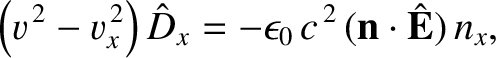can be written as a superposition of sinusoidal waveforms of various different angular frequencies,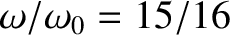. In other words,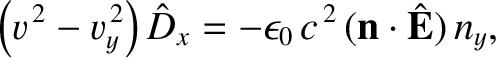(755)

where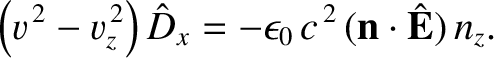and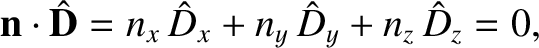are the temporal cosine and sine Fourier transforms of the waveform, respectively. By analogy with Equations (707)-(709), we can invert the above expression to give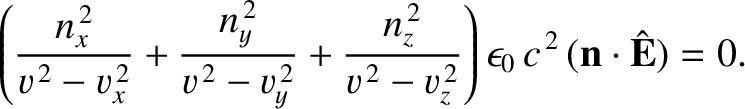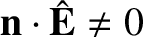(756)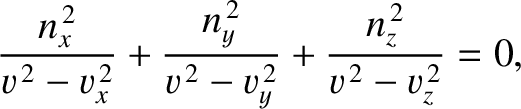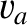(757)

These equations make it manifest that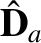, and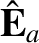. Moreover, it is apparent that ifis an even function of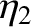then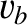, but if it is an odd function then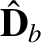.

The current flowing in the antenna of an amplitude-modulated (AM) radio transmitter is driven by a voltage signal that oscillates sinusoidally at a frequency,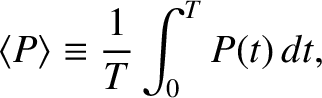, which is known as the carrier frequency. In commercial (medium wave) AM radio, each station is assigned a single carrier frequency that lies somewhere between about 500kHz and 1600kHz. However, the voltage signal fed to the antenna does not have a constant amplitude. Rather, it has a modulated amplitude that can be expressed, somewhat schematically, as a Fourier series: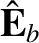(758)

where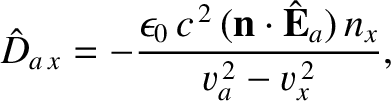represents the information being transmitted. Typically, this information is speech or music which is picked up by a microphone, and converted into an electrical signal. The constant amplitude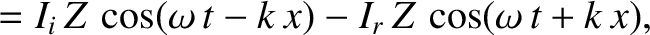is present even when the transmitter is sending no information. The remaining terms in the above expression are due to the signal picked up by the microphone. The modulation frequencies,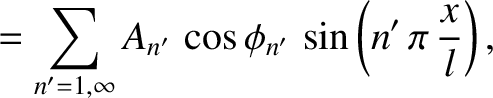, are thus the frequencies of audible sound waves. In other words, they are so-called audio frequencies lying between about 20Hz and 20kHz. This implies that the modulation frequencies are much smaller than the carrier frequency: that is,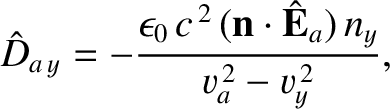for all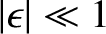. Furthermore, the modulation amplitudes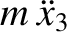are all generally smaller than the carrier amplitude.

The signal transmitted by an AM station, and received by an AM receiver, is an amplitude modulated sinusoidal oscillation of the form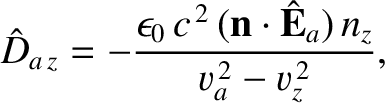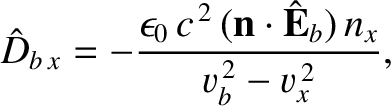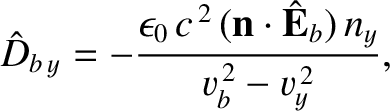(759)

which, with the help of some standard trigonometric identities (see Appendix B), can also be written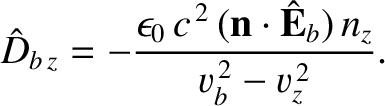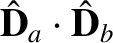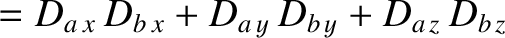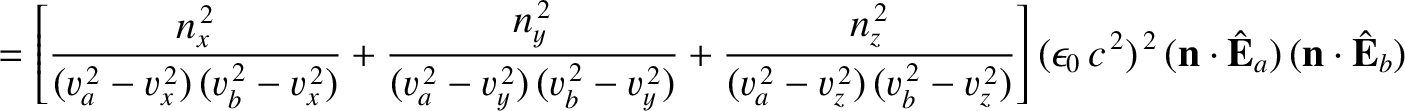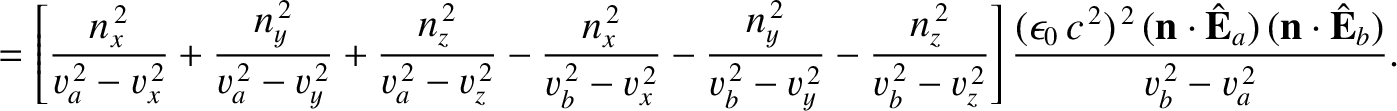(760)

We can calculate the cosine and sine Fourier transforms of the signal,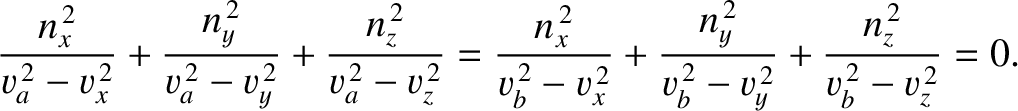(761)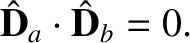(762)

by making use of Equation (727)-(729). It follows that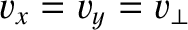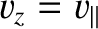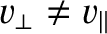(763)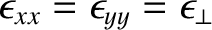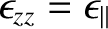(764)

Here, we have only shown the positive frequency components ofand, because we know thatand.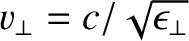The AM frequency spectrum specified in Equations (763) and (764) is shown, somewhat schematically, in Figure 53. The spectrum consists of a series of delta function spikes. The largest spike corresponds to the carrier frequency,. However, this spike carries no information. Indeed, the signal information is carried in so-called sideband frequencies which are equally spaced on either side of the carrier frequency. The upper sidebands correspond to the frequencies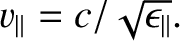, whereas the lower sidebands correspond to the frequencies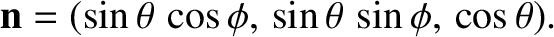. Thus, in order for an AM radio signal to carry all of the information present in audible sound, for which the appropriate modulation frequencies,, range from about 0 Hz to about 20kHz, the signal would have to consist of a superposition of sinusoidal oscillations with frequencies that range from the carrier frequency minus 20kHz to the carrier frequency plus 20kHz. In other words, the signal would have to occupy a range of frequencies from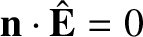to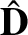, where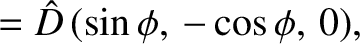is the largest modulation frequency. This is an important result. An AM radio signal that only consists of a single frequency, such as the carrier frequency, transmits no information. Only a signal that occupies a finite range of frequencies, centered on the carrier frequency, is capable of transmitting useful information. The difference between the highest and the lowest frequency components of an AM radio signal, which is twice the maximum modulation frequency, is called the bandwidth of the signal. Thus, to transmit all of the information present in audible sound an AM signal would need to have a bandwidth of 40kHz. In fact, commercial AM radio signals are only allowed to broadcast a bandwidth of 10kHz, in order to maximize the number of available stations. (Two different stations cannot broadcast in frequency ranges that overlap.) This means that commercial AM radio can only carry audible information in the range 0 to about 5kHz. This is perfectly adequate for ordinary speech, but only barely adequate for music.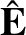Let us now consider how we might transmit a digital signal over AM radio. Suppose that each data bit'' in the signal takes the form of a Gaussian envelope, of characteristic duration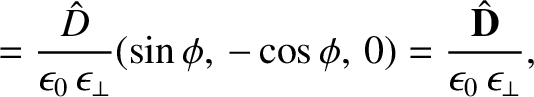, superimposed on a carrier wave whose frequency is: that is,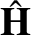(765)

Let us assume that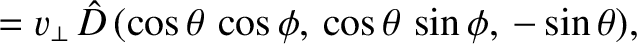. In other words, the period of the carrier wave is much less than the duration of the bit. Figure 54 illustrates a digital bit calculated for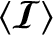.

The sine Fourier transform of the signal (765) is zero by symmetry. However, its cosine Fourier transform takes the form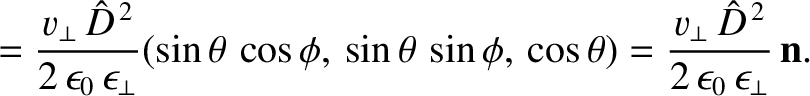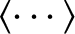(766)

A comparison with Equations (712)-(715) reveals that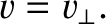(767)

where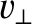(768)

In other words, the Fourier transform of the signal takes the form of a Gaussian in-space, which is centered on the carrier frequency,, and is of characteristic width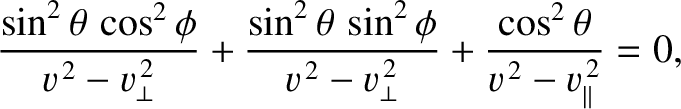. Thus, the bandwidth of the signal is of order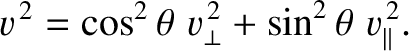. The shorter the signal duration, the higher the bandwidth. This is a general rule. A signal of full width at half maximum temporal duration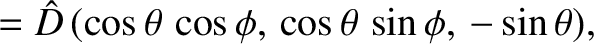generally has a Fourier transform of full width at half maximum bandwidth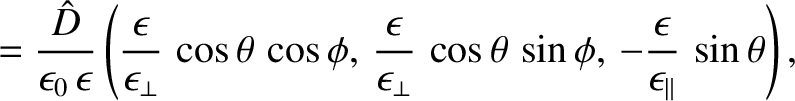, so that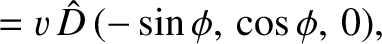(769)

This can also be written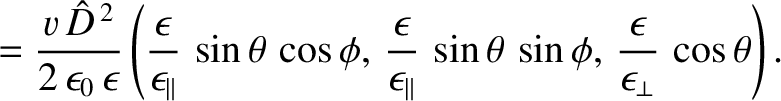(770)

where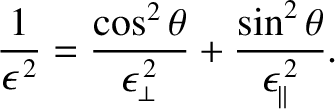is the bandwidth in hertz. The above result is known as the bandwidth theorem. The duration of a digital bit is closely related to the maximum rate at which information can be transmitted by a digital signal. Because the individual bits cannot overlap in time, the maximum number of bits per second that can be transmitted by a digital signal is of order. In other words, it is of order the bandwidth. Thus, digital signals that transmit information at a rapid rate require large bandwidths, and, consequently occupy a wide range of frequency space.

An old-fashioned black and white TV screen consists of a rectangular grid of black and white spots scanned by an electron beam. A given spot is white'' if the phosphorescent TV screen was recently (i.e., within aboutth of a second) struck by the electron beam at that location. The spot separation is about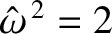mm. A typical screen is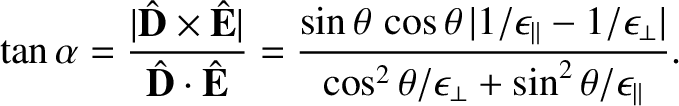, and thus has 500 lines with 500 spots per line, or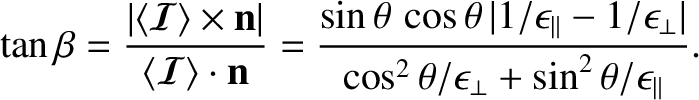spots. Each spot is renewed every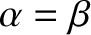th of a second. (Every other horizontal line is skipped during a given traversal of the electron beam over the screen. The skipped lines are renewed on the next traversal. This technique is known as interlacing. Consequently, a given region of the screen, that includes many horizontal lines, has a flicker rate of 60 Hz.) Thus, the rate at which the instructions turn on" and turn off'' must be sent to the electron beam is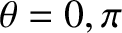or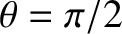times a second. The transmitted TV signal must therefore have about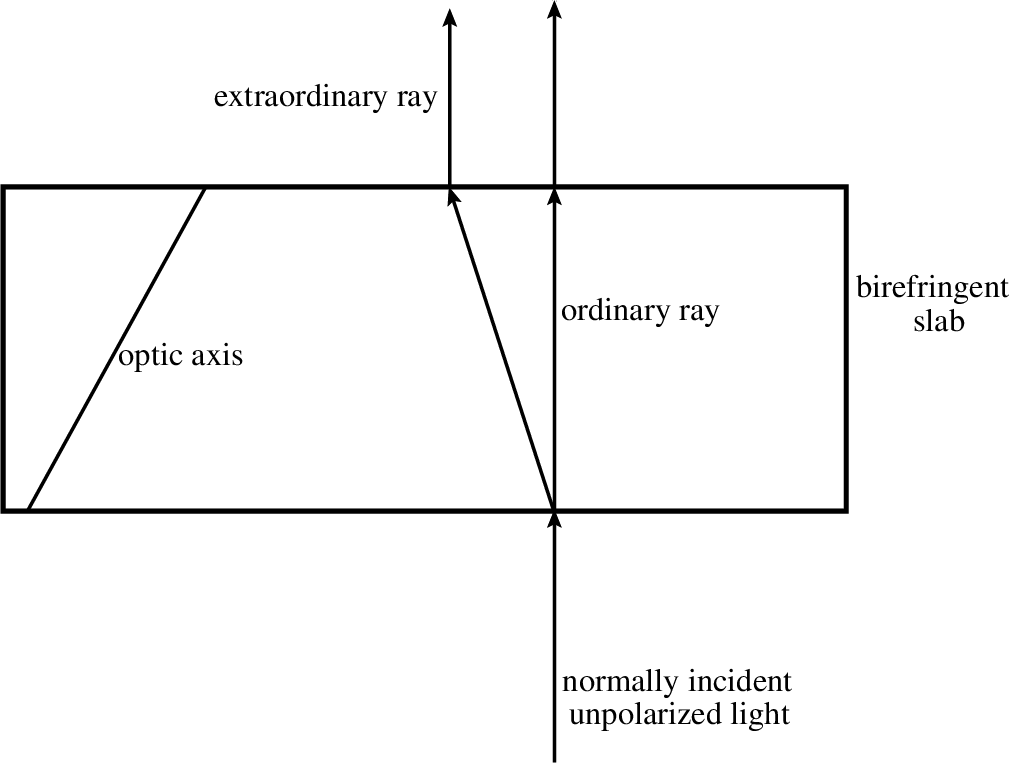on-off instruction blips per second. If temporal overlap is to be avoided, each blip can be no longer than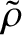seconds in duration. Thus, the required bandwidth is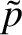. The carrier wave frequencies used for conventional broadcast TV lie in the so-called VHF band, and range from about 55 to 210 MHz. Our previous discussion of AM radio might lead us to think that the 10MHz bandwidth represents the combined extents of an upper and a lower sideband of modulation frequencies. In practice, the carrier wave and one of the sidebands are suppressed. That is, they are filtered out, and never applied to the antenna. However, they are regenerated in the receiver from the information contained in the single sideband that is broadcast. This technique, which is called single sideband transmission, halves the bandwidth requirement to about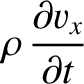. (Incidentally, the lower sideband carries the same information as the upper one, and thus can be used to completely regenerate the upper sideband, and vice versa.) Thus, between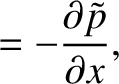and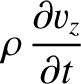there is room for about 30 TV channels, each using a 5MHz bandwidth. (Actually, there are far fewer TV channels than this in the VHF band, because part of this band is reserved for FM radio, air traffic control, air navigation beacons, marine communications, etc.)Next: Exercises Up: Wave Pulses Previous: General Solution of 1D
Richard Fitzpatrick 2013-04-08